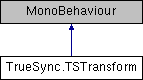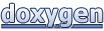# TrueSync: TrueSync.TSTransform Class Reference

#### TrueSync

TrueSync.TSTransform Class Reference

A deterministic version of Unity's Transform component for 3D physics. More...

Inheritance diagram for TrueSync.TSTransform:## Public Member Functions

void LookAt (TSTransform other)
Rotates game object to point forward vector to a target position. More...

void LookAt (TSVector target)
Rotates game object to point forward vector to a target position. More...

void Translate (FP x, FP y, FP z)
Moves game object based on provided axis values.

void Translate (FP x, FP y, FP z, Space relativeTo)
Moves game object based on provided axis values and a relative space. More...

void Translate (FP x, FP y, FP z, TSTransform relativeTo)
Moves game object based on provided axis values and a relative TSTransform. More...

void Translate (TSVector translation)
Moves game object based on provided translation vector.

void Translate (TSVector translation, Space relativeTo)
Moves game object based on provided translation vector and a relative space. More...

void Translate (TSVector translation, TSTransform relativeTo)
Moves game object based on provided translation vector and a relative TSTransform. More...

void RotateAround (TSVector point, TSVector axis, FP angle)
Rotates game object based on provided axis, point and angle of rotation.

void RotateAround (TSVector axis, FP angle)
Rotates game object based on provided axis and angle of rotation.

void Rotate (FP xAngle, FP yAngle, FP zAngle)
Rotates game object based on provided axis angles of rotation.

void Rotate (FP xAngle, FP yAngle, FP zAngle, Space relativeTo)
Rotates game object based on provided axis angles of rotation and a relative space. More...

void Rotate (TSVector eulerAngles)
Rotates game object based on provided axis angles of rotation.

void Rotate (TSVector axis, FP angle)
Rotates game object based on provided axis and angle of rotation.

void Rotate (TSVector axis, FP angle, Space relativeTo)
Rotates game object based on provided axis, angle of rotation and relative space. More...

void Rotate (TSVector eulerAngles, Space relativeTo)
Rotates game object based on provided axis angles and relative space. More...

void Start ()

void Initialize ()
Initializes internal properties based on whether there is a TSCollider attached.

void Update ()

## Public Attributes

TSCollider tsCollider

TSTransform tsParent

## Properties

TSVector position` [get, set]`

TSQuaternion rotation` [get, set]`

TSVector scale` [get, set]`

TSVector forward` [get]`
Current self forward vector.

TSVector right` [get]`
Current self right vector.

TSVector up` [get]`
Current self up vector.

TSVector eulerAngles` [get]`
Returns Euler angles in degrees.

## Detailed Description

A deterministic version of Unity's Transform component for 3D physics.

Definition at line 9 of file TSTransform.cs.

## Member Function Documentation

 void TrueSync.TSTransform.LookAt ( TSTransform other )

Rotates game object to point forward vector to a target position.

Parameters
 other TSTrasform used to get target position.

Definition at line 103 of file TSTransform.cs.

 void TrueSync.TSTransform.LookAt ( TSVector target )

Rotates game object to point forward vector to a target position.

Parameters
 target Target position.

Definition at line 112 of file TSTransform.cs.

 void TrueSync.TSTransform.Rotate ( FP xAngle, FP yAngle, FP zAngle, Space relativeTo )

Rotates game object based on provided axis angles of rotation and a relative space.

If relative space is SELF then the game object will rotate based on its forward vector.

Definition at line 202 of file TSTransform.cs.

 void TrueSync.TSTransform.Rotate ( TSVector axis, FP angle, Space relativeTo )

Rotates game object based on provided axis, angle of rotation and relative space.

If relative space is SELF then the game object will rotate based on its forward vector.

Definition at line 225 of file TSTransform.cs.

 void TrueSync.TSTransform.Rotate ( TSVector eulerAngles, Space relativeTo )

Rotates game object based on provided axis angles and relative space.

If relative space is SELF then the game object will rotate based on its forward vector.

Definition at line 243 of file TSTransform.cs.

 void TrueSync.TSTransform.Translate ( FP x, FP y, FP z, Space relativeTo )

Moves game object based on provided axis values and a relative space.

If relative space is SELF then the game object will move based on its forward vector.

Definition at line 128 of file TSTransform.cs.

 void TrueSync.TSTransform.Translate ( FP x, FP y, FP z, TSTransform relativeTo )

Moves game object based on provided axis values and a relative TSTransform.

The game object will move based on TSTransform's forward vector.

Definition at line 137 of file TSTransform.cs.

 void TrueSync.TSTransform.Translate ( TSVector translation, Space relativeTo )

Moves game object based on provided translation vector and a relative space.

If relative space is SELF then the game object will move based on its forward vector.

Definition at line 153 of file TSTransform.cs.

 void TrueSync.TSTransform.Translate ( TSVector translation, TSTransform relativeTo )

Moves game object based on provided translation vector and a relative TSTransform.

The game object will move based on TSTransform's forward vector.

Definition at line 166 of file TSTransform.cs.

## Property Documentation

 TSVector TrueSync.TSTransform.position
getset

It works as proxy to a Body's position when there is a collider attached.

Definition at line 23 of file TSTransform.cs.

 TSQuaternion TrueSync.TSTransform.rotation
getset

Generated by1.8.11